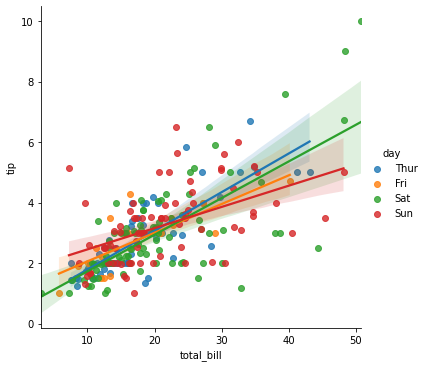# Data Visualization With Seaborn - Part 1

In this blog we will see scatter plotting and different type of category plotting.

## What is Seaborn?

Seaborn is a Python data visualization library based on matplotlib. It provides a high-level interface for drawing attractive and informative statistical graphics.

## Installing and getting started

seaborn can be installed from PyPI.

### Required dependencies

numpy

scipy

pandas

matplotlib

Open command prompt in your system and install seaborn library.

pip install seaborn

The library is also included as part of the Anaconda distribution:

conda install seaborn

## Import Libraries

In :
import seaborn as sns
import matplotlib.pyplot as plt


dir(sns)

## Load an example dataset from the online repository

### View the dataset available with seaborn library

In :
sns.get_dataset_names()

Out:
['anagrams',
'anscombe',
'attention',
'brain_networks',
'car_crashes',
'diamonds',
'dots',
'exercise',
'flights',
'fmri',
'gammas',
'geyser',
'iris',
'mpg',
'penguins',
'planets',
'tips',
'titanic']

Some sample datasets are available with seaborn library. Let us take one database "tips" and plot some graph.

In :
tips = sns.load_dataset('tips')
tips

Out:
total_bill tip sex smoker day time size
0 16.99 1.01 Female No Sun Dinner 2
1 10.34 1.66 Male No Sun Dinner 3
2 21.01 3.50 Male No Sun Dinner 3
3 23.68 3.31 Male No Sun Dinner 2
4 24.59 3.61 Female No Sun Dinner 4
... ... ... ... ... ... ... ...
239 29.03 5.92 Male No Sat Dinner 3
240 27.18 2.00 Female Yes Sat Dinner 2
241 22.67 2.00 Male Yes Sat Dinner 2
242 17.82 1.75 Male No Sat Dinner 2
243 18.78 3.00 Female No Thur Dinner 2

244 rows × 7 columns

sns.set(color_codes=True)

## Plot scatter plot

### seaborn.scatterplot

seaborn.scatterplot(*, x=None, y=None, hue=None, style=None, size=None, data=None, palette=None, hue_order=None, hue_norm=None, sizes=None, size_order=None, size_norm=None, markers=True, style_order=None, x_bins=None, y_bins=None, units=None, estimator=None, ci=95, n_boot=1000, alpha=None, x_jitter=None, y_jitter=None, legend='auto', ax=None, **kwargs)

Draw a scatter plot with possibility of several semantic groupings.



The relationship between x and y can be shown for different subsets of the data using the hue, size, and style parameters. These parameters control what visual semantics are used to identify the different subsets.

#### Parameters

x, y: vectors or keys in data

Variables that specify positions on the x and y axes.

hue: vector or key in data

Grouping variable that will produce points with different colors. Can be either categorical or numeric, although color mapping will behave differently in latter case.

size: vector or key in data

Grouping variable that will produce points with different sizes. Can be either categorical or numeric, although size mapping will behave differently in latter case.

style: vector or key in data

Grouping variable that will produce points with different markers. Can have a numeric dtype but will always be treated as categorical.

data: pandas.DataFrame, numpy.ndarray, mapping, or sequence

Input data structure. Either a long-form collection of vectors that can be assigned to named variables or a wide-form dataset that will be internally reshaped.

palette: string, list, dict, or matplotlib.colors.Colormap

Method for choosing the colors to use when mapping the hue semantic. String values are passed to color_palette(). List or dict values imply categorical mapping, while a colormap object implies numeric mapping.

hue_orde: rvector of strings

Specify the order of processing and plotting for categorical levels of the hue semantic.

hue_norm: tuple or matplotlib.colors.Normalize

Either a pair of values that set the normalization range in data units or an object that will map from data units into a [0, 1] interval. Usage implies numeric mapping.

sizes: list, dict, or tuple

An object that determines how sizes are chosen when size is used. It can always be a list of size values or a dict mapping levels of the size variable to sizes. When size is numeric, it can also be a tuple specifying the minimum and maximum size to use such that other values are normalized within this range.

size_order: list

Specified order for appearance of the size variable levels, otherwise they are determined from the data. Not relevant when the size variable is numeric.

size_norm: tuple or Normalize object

Normalization in data units for scaling plot objects when the size variable is numeric.

markers: boolean, list, or dictionary

Object determining how to draw the markers for different levels of the style variable. Setting to True will use default markers, or you can pass a list of markers or a dictionary mapping levels of the style variable to markers. Setting to False will draw marker-less lines. Markers are specified as in matplotlib.

style_order: list

Specified order for appearance of the style variable levels otherwise they are determined from the data. Not relevant when the style variable is numeric.

{x,y}_bins: lists or arrays or functions

Currently non-functional.

units: vector or key in data

Grouping variable identifying sampling units. When used, a separate line will be drawn for each unit with appropriate semantics, but no legend entry will be added. Useful for showing distribution of experimental replicates when exact identities are not needed. Currently non-functional.
estimatorname of pandas method or callable or None

Method for aggregating across multiple observations of the y variable at the same x level. If None, all observations will be drawn. Currently non-functional.

ci: int or “sd” or None

Size of the confidence interval to draw when aggregating with an estimator. “sd” means to draw the standard deviation of the data. Setting to None will skip bootstrapping. Currently non-functional.

n_boot: int

Number of bootstraps to use for computing the confidence interval. Currently non-functional.

alpha: float

Proportional opacity of the points.

{x,y}_jitter: booleans or floats

Currently non-functional.

legend: “auto”, “brief”, “full”, or False

How to draw the legend. If “brief”, numeric hue and size variables will be represented with a sample of evenly spaced values. If “full”, every group will get an entry in the legend. If “auto”, choose between brief or full representation based on number of levels. If False, no legend data is added and no legend is drawn.

ax: matplotlib.axes.Axes

Pre-existing axes for the plot. Otherwise, call matplotlib.pyplot.gca() internally.

kwargs: key, value mappings

Other keyword arguments are passed down to matplotlib.axes.Axes.scatter().



#### Returns

matplotlib.axes.Axes

The matplotlib axes containing the plot.
In :
sns.scatterplot(x = 'total_bill', y = 'tip', data = tips)

Out:
<AxesSubplot:xlabel='total_bill', ylabel='tip'>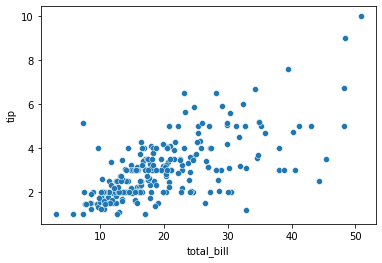hue : It will produce data points with different colors.

In :
sns.scatterplot(x = "total_bill", y = "tip", hue = "day", data = tips)

Out:
<AxesSubplot:xlabel='total_bill', ylabel='tip'>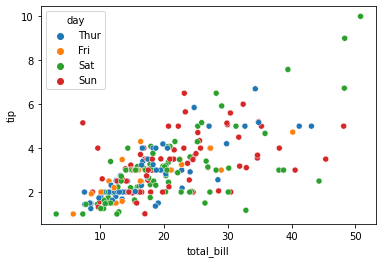style: Pass value as a name of variables or vector from DataFrame, it will group variable and produce points with different markers.

In :
sns.scatterplot(x = "total_bill", y = "tip", hue = "day", style = "time", data = tips)

Out:
<AxesSubplot:xlabel='total_bill', ylabel='tip'>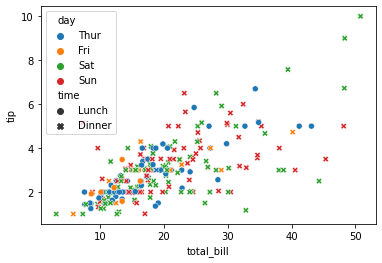## Categorical scatterplots

In :
sns.catplot(x = "day", y = "total_bill", data = tips)

Out:
<seaborn.axisgrid.FacetGrid at 0x7f14f1a9fb10>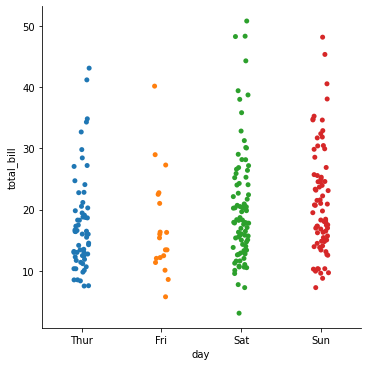The jitter parameter controls the magnitude of jitter or disables it altogether:

In :
sns.catplot(x = "day", y = "total_bill", jitter = False, data = tips)

Out:
<seaborn.axisgrid.FacetGrid at 0x7f14f1a51090>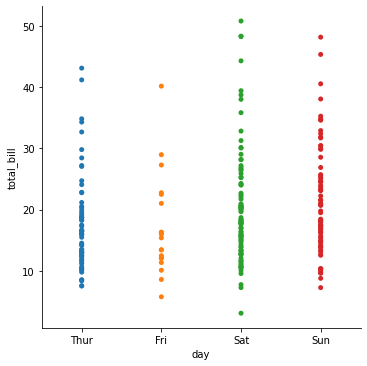The second approach adjusts the points along the categorical axis using an algorithm that prevents them from overlapping. It can give a better representation of the distribution of observations.

This kind of plot is sometimes called a “beeswarm” and is drawn in seaborn by swarmplot(), which is activated by setting kind="swarm" in catplot():

In :
sns.catplot(x = "day", y = "total_bill", kind = "swarm", data = tips)

Out:
<seaborn.axisgrid.FacetGrid at 0x7f14f1a70690>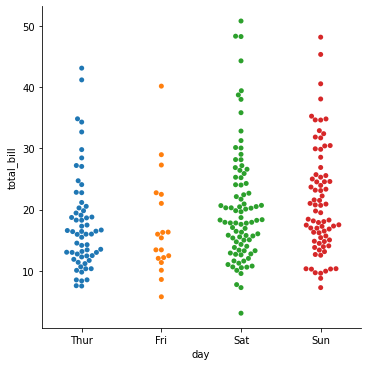### Add another dimension to a categorical plot by using a hue semantic

Each different categorical plotting function handles the hue semantic differently.

In :
sns.catplot(x = "day", y = "total_bill", hue = "sex", kind = "swarm", data = tips)

Out:
<seaborn.axisgrid.FacetGrid at 0x7f14f1c5ef10>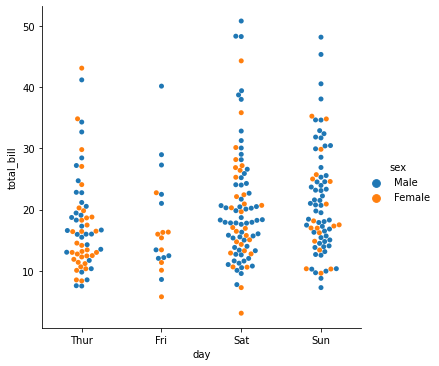In :
sns.catplot(x = "day", y = "total_bill", hue = "smoker", kind = "swarm", data = tips);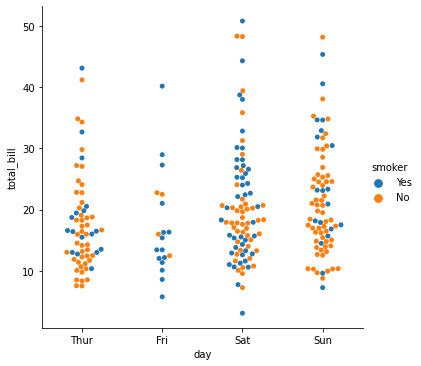Filter size column data and then plot

In :
sns.catplot(x = "size", y = "total_bill", kind = "swarm", data = tips.query("size != 3"));

/opt/tljh/user/lib/python3.7/site-packages/seaborn/categorical.py:1296: UserWarning: 7.7% of the points cannot be placed; you may want to decrease the size of the markers or use stripplot.
warnings.warn(msg, UserWarning)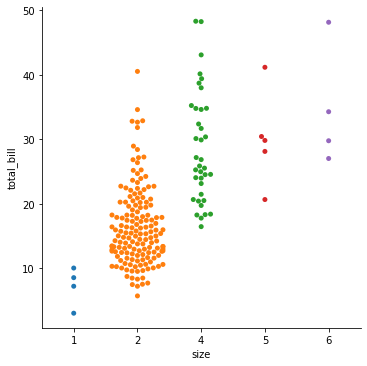### Categorical plot with box ploting

In :
sns.catplot(x = "day", y = "total_bill", kind = "box", data = tips)

Out:
<seaborn.axisgrid.FacetGrid at 0x7f14f1931310>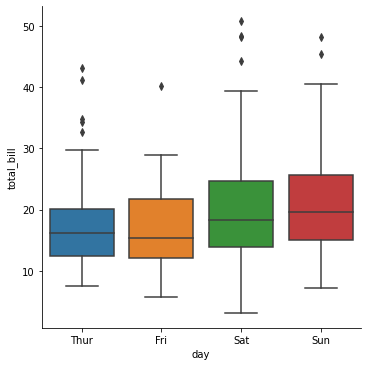### Add hue parameter and legend in box plotting

In :
g = sns.catplot(x = "day", y = "total_bill",  hue = "time", kind = 'box', data = tips)
plt.show()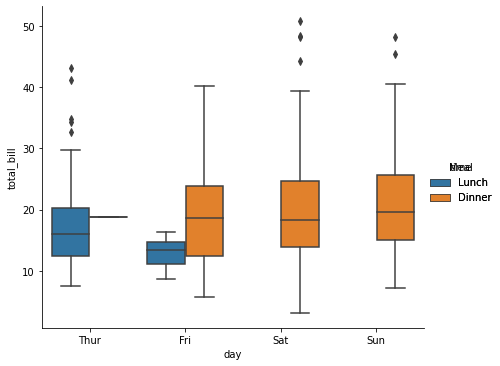### Set the x and y axis label

In :
g = sns.catplot(x = "day", y = "total_bill",  hue = "time", kind = 'box', data = tips)
g.set_axis_labels("", "Total bill ($)")  Out: <seaborn.axisgrid.FacetGrid at 0x7f14eb76a490>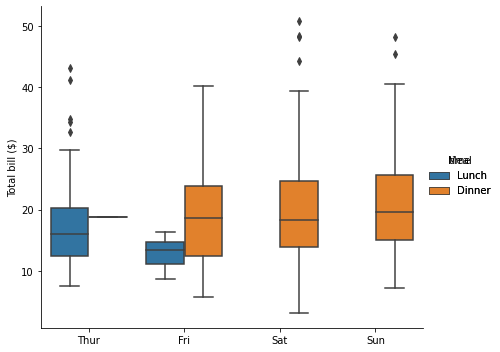### Increase or decrease the size of a matplotlib plot To increase or decrease the size of a matplotlib plot, you set the width and height of the entire figure, either in the global rcParams, while setting up the plot (e.g. with the figsize parameter of matplotlib.pyplot.subplots()), or by calling a method on the figure object (e.g. matplotlib.Figure.set_size_inches()). m In : g = sns.catplot(x = "day", y = "total_bill", hue = "time", kind = 'box', data = tips) g.add_legend(title = "Meal") g.fig.set_size_inches(10.5, 5.5) g.set_axis_labels("", "Total bill ($)")

Out:
<seaborn.axisgrid.FacetGrid at 0x7f14f1663050>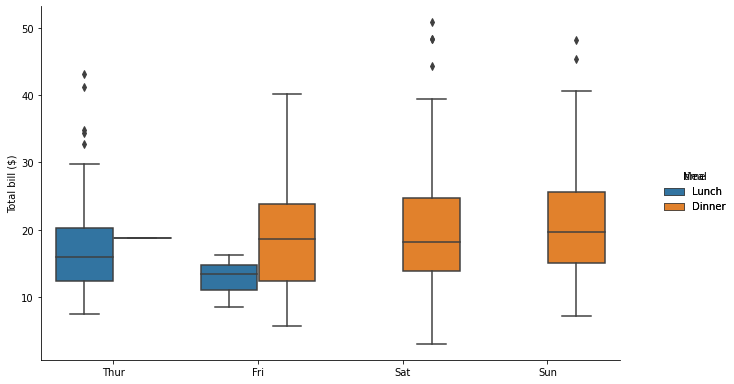In :
g = sns.catplot(x = "day", y = "total_bill",  hue = "time",
height = 3.5, aspect = 1.5, kind = 'box', data = tips)

g.set_axis_labels("", "Total bill (\$)")

g.set(ylim = (0, 60), xticklabels = ["Thursday", "Friday", "Saturday", "Sunday"])

g.fig.set_size_inches(12.5, 8.5)
g.ax.set_yticks([5, 15, 25, 35, 45, 55], minor = True);
plt.setp(g.ax.get_xticklabels(), rotation=30);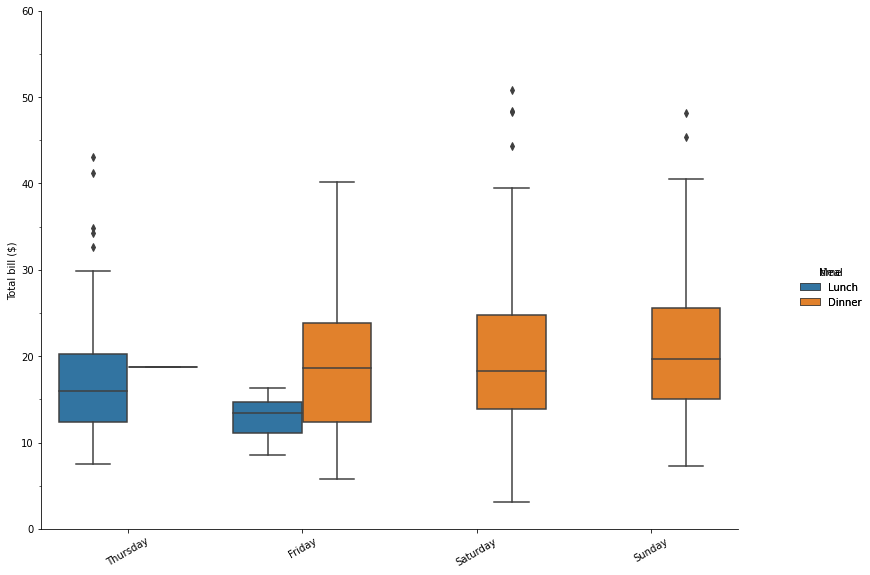## Some other categorical plots

### Violin plot

In :
sns.catplot(x = "day", y = "total_bill", hue = "smoker", kind = "violin", data = tips);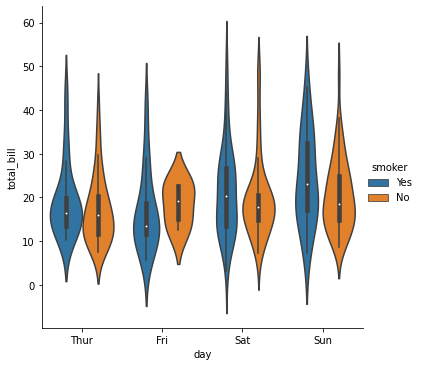### Bar plotting with categorical plot

In :
sns.catplot(x = "day", y = "total_bill", hue = "smoker", kind = "bar", data = tips);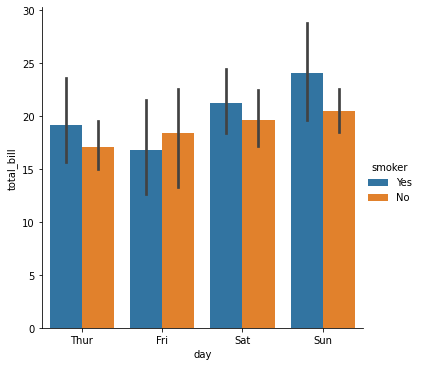### Boxen plotting

In :
g = sns.catplot(x = "day", y = "total_bill", hue = "time", kind = "boxen", data = tips);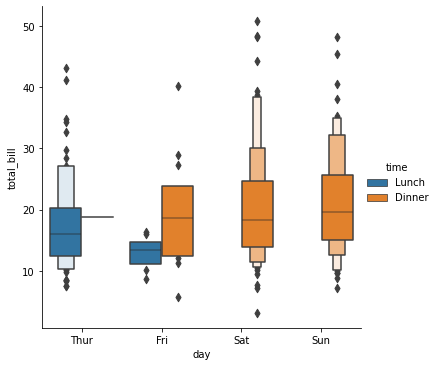## Plot data with regression line

seaborn.lmplot: Plot data and regression model fits across a FacetGrid.

to enhance a scatterplot to include a linear regression model (and its uncertainty) using lmplot():

In :
sns.lmplot(x = "total_bill", y = "tip", data = tips)

Out:
<seaborn.axisgrid.FacetGrid at 0x7f14f17b6cd0>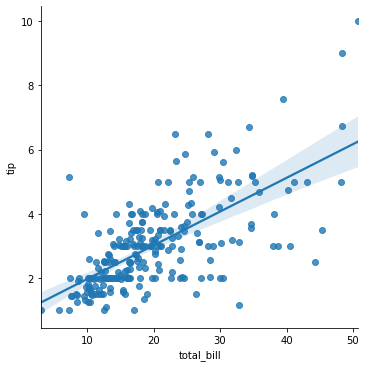In :
sns.lmplot(x = "total_bill", y = "tip", data = tips, hue = "time")

Out:
<seaborn.axisgrid.FacetGrid at 0x7f14f1310f10>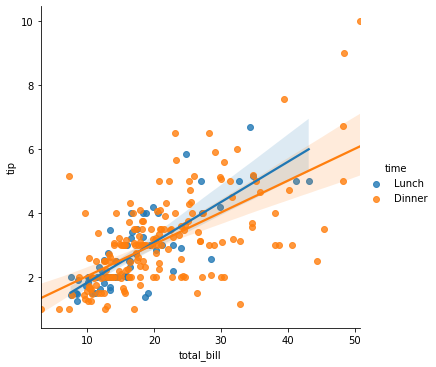In :
sns.lmplot(x = "total_bill", y = "tip", data = tips, hue="day")

Out:
<seaborn.axisgrid.FacetGrid at 0x7f14f0224110>